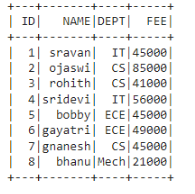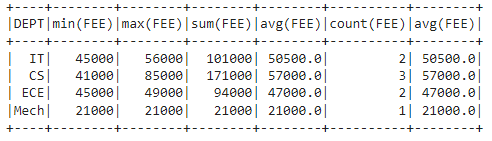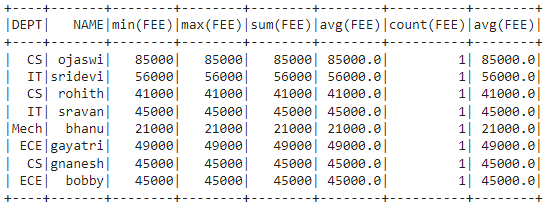GeeksforGeeks App
Open AppBrowser
Continue

# Multiple criteria for aggregation on PySpark Dataframe

In this article, we will discuss how to do Multiple criteria aggregation on PySpark Dataframe.

### Data frame in use:In PySpark,  groupBy() is used to collect the identical data into groups on the PySpark DataFrame and perform aggregate functions on the grouped data. So by this we can do multiple aggregations at a time.

Syntax:

dataframe.groupBy(‘column_name_group’).agg(functions)

where,

• column_name_group is the column to be grouped
• functions are the aggregation functions

Lets understand what are the aggregations first. They are available in functions module in pyspark.sql, so we need to import it to start with. The aggregate functions are:

• count(): This will return the count of rows for each group.

Syntax:

functions.count(‘column_name’)

• mean(): This will return the mean of values for each group.

Syntax:

functions.mean(‘column_name’)

• max(): This will return the maximum of values for each group.

Syntax:

functions.max(‘column_name’)

• min(): This will return the minimum of values for each group.

Syntax:

functions.min(‘column_name’)

• sum(): This will return the total values for each group.

Syntax:

functions.sum(‘column_name’)

• avg(): This will return the average for values for each group.

Syntax:

functions.avg(‘column_name’)

We can aggregate multiple functions using the following syntax.

Syntax:

dataframe.groupBy(‘column_name_group’).agg(functions….)

Example: Multiple aggregations on DEPT column with FEE column

## Python3

 `# importing module``import` `pyspark`` ` `# importing sparksession from pyspark.sql module``from` `pyspark.sql ``import` `SparkSession`` ` `#import functions``from` `pyspark.sql ``import` `functions`` ` `# creating sparksession and giving an app name``spark ``=` `SparkSession.builder.appName(``'sparkdf'``).getOrCreate()`` ` `# list  of student  data``data ``=` `[[``"1"``, ``"sravan"``, ``"IT"``, ``45000``],``        ``[``"2"``, ``"ojaswi"``, ``"CS"``, ``85000``],``        ``[``"3"``, ``"rohith"``, ``"CS"``, ``41000``],``        ``[``"4"``, ``"sridevi"``, ``"IT"``, ``56000``],``        ``[``"5"``, ``"bobby"``, ``"ECE"``, ``45000``],``        ``[``"6"``, ``"gayatri"``, ``"ECE"``, ``49000``],``        ``[``"7"``, ``"gnanesh"``, ``"CS"``, ``45000``],``        ``[``"8"``, ``"bhanu"``, ``"Mech"``, ``21000``]``        ``]`` ` `# specify column names``columns ``=` `[``'ID'``, ``'NAME'``, ``'DEPT'``, ``'FEE'``]`` ` `# creating a dataframe from the lists of data``dataframe ``=` `spark.createDataFrame(data, columns)`` ` ` ` `# aggregating DEPT column with min.max,sum,mean,avg and count functions``dataframe.groupBy(``'DEPT'``).agg(functions.``min``(``'FEE'``),``                              ``functions.``max``(``'FEE'``),``                              ``functions.``sum``(``'FEE'``), ``                              ``functions.mean(``'FEE'``),``                              ``functions.count(``'FEE'``),``                              ``functions.avg(``'FEE'``)).show()`

Output:Example 2: Multiple aggregation in grouping dept and name column

## Python3

 `# importing module``import` `pyspark`` ` `# importing sparksession from pyspark.sql module``from` `pyspark.sql ``import` `SparkSession`` ` `#import functions``from` `pyspark.sql ``import` `functions`` ` `# creating sparksession and giving an app name``spark ``=` `SparkSession.builder.appName(``'sparkdf'``).getOrCreate()`` ` `# list  of student  data``data ``=` `[[``"1"``, ``"sravan"``, ``"IT"``, ``45000``],``        ``[``"2"``, ``"ojaswi"``, ``"CS"``, ``85000``],``        ``[``"3"``, ``"rohith"``, ``"CS"``, ``41000``],``        ``[``"4"``, ``"sridevi"``, ``"IT"``, ``56000``],``        ``[``"5"``, ``"bobby"``, ``"ECE"``, ``45000``],``        ``[``"6"``, ``"gayatri"``, ``"ECE"``, ``49000``],``        ``[``"7"``, ``"gnanesh"``, ``"CS"``, ``45000``],``        ``[``"8"``, ``"bhanu"``, ``"Mech"``, ``21000``]``        ``]`` ` `# specify column names``columns ``=` `[``'ID'``, ``'NAME'``, ``'DEPT'``, ``'FEE'``]`` ` `# creating a dataframe from the lists of data``dataframe ``=` `spark.createDataFrame(data, columns)`` ` ` ` `# aggregating DEPT, NAME column with min.max,``# sum,mean,avg and count functions``dataframe.groupBy(``'DEPT'``, ``'NAME'``).agg(functions.``min``(``'FEE'``), ``                                      ``functions.``max``(``'FEE'``), ``                                      ``functions.``sum``(``'FEE'``),``                                      ``functions.mean(``'FEE'``), ``                                      ``functions.count(``'FEE'``), ``                                      ``functions.avg(``'FEE'``)).show()`

Output:My Personal Notes arrow_drop_up